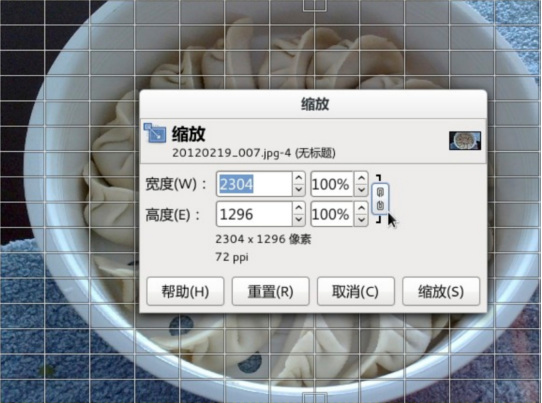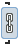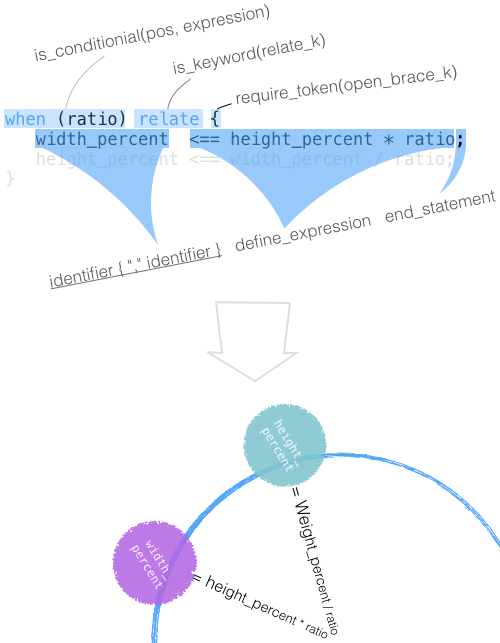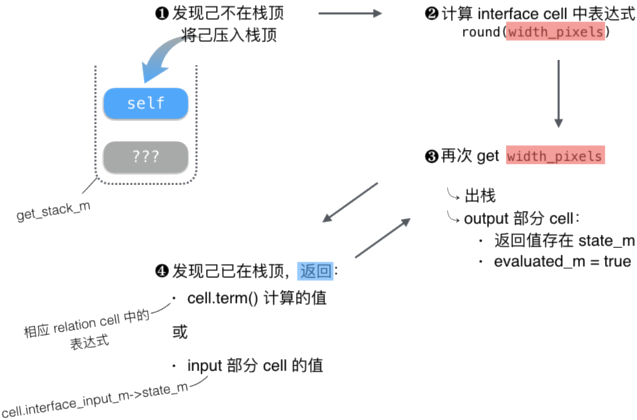微信公众号 关注我们 知识星球关注 @泰晓科技与数百位一线 Linux 工程师做朋友，您准备好了吗？分享 Linux 产品实战经验。 与业界 Linux 专家交流。# 量子化的 UI

 微信公众号 知识星球关注 @泰晓科技与数百位一线 Linux 工程师做朋友，您准备好了吗？分享 Linux 产品实战经验。 与业界 Linux 专家交流。

By Chen Jie of TinyLab.org 2016-01-19 21:07:18

## 引言

2015.11 第三期的泰晓资讯中，提到了 LWN 转载文章「No UI is some UI」。同时，本站「移动终端 UI 设计新思路：从图形界面回归“命令行”?」 提出了聊天风格主导的手机系统界面。用户通过 简单格式的语句，或甚至是自然语言，来完成交互。而「大开脑洞，做一个比表屏大，比 phone 便携的移动设备」一文， 展示了 输入法协助用户生成指令 ，来方便聊天风格的交互。（如何用聊天风格 UI 来满足业务交互？贩一个“私货”：Logging and Tickets。通过交互逐步确定具体的目标，交互过程叫做“Logging”，确定下来的目标叫做“Tickets”。比如，准备出国游去移动营业厅开通“全球漫游服务”，与服务窗口 mm 交谈为 Logging；最后开通具体业务，落款的合同为 Ticket。通过 “Logging”，可回溯细节，并智能化推荐和协助。而 Tickets 则按不同线归档 /Ticket Book/，例如按时间线、位置线归档，从而智能化呈现）

Definition: A User Interface (UI) is a system for assisting a user in selecting a function and providing a valid set of parameters to the function.

Definition: A Graphical User Interface (GUI) is a visual and interactive UI.

Sean Parent, 「Adobe Source Libraries Overview & Philosophy」, p49## Adam ∙ 例子``````sheet scale_image {
input:
ratio             : 0.0;
original_width    : 2304;
original_height   : 1296;
interface:
width_pixels      : original_width  <== round(width_pixels);
width_percent;
height_pixels     : original_height <== round(height_pixels);
height_percent;
logic:
relate {
width_pixels   <== round(width_percent * original_width / 100);
width_percent  <== width_pixels * 100 / original_width;
}

relate {
height_pixels  <== round(height_percent * original_height / 100);
height_percent <== height_pixels * 100 / original_height;
}

when (ratio) relate {
width_percent  <== height_percent * ratio;
height_percent <== width_percent / ratio;
}
output:
result <== { height: height_pixels, width: width_pixels };
}
``````

``````relate {
width_pixels   <== round(width_percent * original_width / 100);
width_percent  <== width_pixels * 100 / original_width;
}
``````

• 用户填入缩放后宽度（width_pixels）⟶ width_percent 重新计算。
• 用户点击锁定宽高比：（set ratio = width_percent / height_percent）。用户再更新 width_pixels ⟶ width_percent ⟶ height_percent ⟶ height_pixels 依次重新计算。

cell 的类型参见附录

## Adam ∙ 内流透视

### 解析（Parsing）``````is_or_expression   // and_expression { "||" and_expression }
^^^^^^^^^^^^^^
is_and_expression   // bitwise_or_expression { "&&" bitwise_or_expression }
^^^^^^^^^^^^^^^^^^^^^
is_bitwise_or_expression   // bitwise_xor_expression { "|" bitwise_xor_expression }

...

is_postfix_expression   // primary_expression { ("[" expression "]") | ("." identifier) }
^^^^^^^^^^^^^^^^^^
is_primary_expression   // name | number | boolean | string | "empty" |
// array | dictionary | variable_or_fuction |
// ( "(" expression ")" )
``````### 计算 cell 之值

• 对于 input 部分的 cell，其值存于成员变量 state_m，或由 sheet_t::implementation_t::set() 来设置；或由构造时，初始化表达式计算而来（函数路径：add_interface()/initialize_one()）。该值可能参与：
• ouput 部分的值计算（见下点）。
• 其他 interface cell 的初值计算。例如，若存在 interface cell `width_dpi: width_pixels * 48 / SCREEN_DPI`，计算初始化表达式时，width_pixels 取初值 “original_width，即 2304”。
• 对于 output 部分的 cell，其值存于成员变量 state_m，在 sheet_t::implementation_t::get() 中计算得：• 通常而言，当 output 部分 cell 值更新后，input 部分 cell 值也会同步成该值，除非指定了 “unlink” 关键字。

### 更新 sheet

1. 处理“有条件的 relation cell(s)”，即 “when(p) relate { … }”。计算条件中的表达式 p，将前提条件不成立的 relation cell(s)，全部标记为已解决（resolved_m = true）。

2. flow() ，前述 `get()` 示意中步骤 ❹ 中的 term_m 在此阶段确立。

3. 遍历 `output_index_m`，对于表达式未被计算的 cells（!evaluated_m），计算之（计算出的值存于 state_m，evaluated_m 置为 true），对于 interface cell(s)，还会同步 input 和 ouput 部分 cell 的值。

4. 遍历 `invariant_index_m`，同上进行表达式计算。当算出的布尔值为假，所有参与计算的 cells 被标记为有毒（poison）。

5. 再次遍历 `output_index_m`，对有变化的 cell(s) 调用通知函数：
• 无效状态发生变化的：例如，原值有效，现参与值计算的某个 cell 有毒，于是变为无效。
• 原值 与 现值 不相等。
• 参与计算的 cell(s) 与上次不同（TODO）。
6. 通过 sheet_t::implementation_t::monitor_enabled 监视的 “input”/“interface (input)” cell，其启用状态发生变化，调用通知函数。以下条件认为 cell 启用：
• 在第二步 flow() 中，访问过其优先级（priority）。
• 或参与计算 output cell 的值（对于 interface cell，注意须是 input 部分参与）。
• 参与计算其他 cell 的值，且参数指定的一组 cell(s) 之优先级被访问过。

### flow()• 对两个 relation cells，有`resolved_m == true`
• `width_percent.relation_count_m == 0`

1. 首先由尚未 resolved relation cells，“收集圈上的 interface cells”，并按照优先级排序：width_pixels > height_percent > height_pixels > width_percent。
2. 按照优先级遍历上述诸 interface cells：
1. 标记 width_pixels.resolved_m = true，表示在 flow 中，本 cell 自身事务已了。
2. width_pixels 所在 relation
• `--width_pixels.relation_count_m`，代表 width_pixels 不再“阻碍”此 relation 的解决，故 “阻碍 relations 们计数”减少。
• 这是一个两元关系，width_pixels 已知（resolved），于是 width_percent.term_m 被确定下来，为 “width_pixels * 100 / original_width”。
• 同时`--width_percent.relation_count_m`、本 relation 也被解决（标记 relsoved_m = true）。
3. 标记 width_percent.resolved_m = true注：这是 width_pixels 更新引起的“涟漪”，故动态调整了优先排序 —— 这种优先级因输入输出而产生动态流动传递，是否对调度器也有所启发呢？
4. width_percent 所在（未解决）relation
• `--width_percent.relation_count_m`
• 同样是一个两元关系，width_percent 已知（resolved），于是 height_percent.term_m 被确定，为 “width_percent / ratio”。
• 同时`--height_percent.relation_count_m`、本 relation 也被解决（标记 relsoved_m = true）。
5. 标记 height_percent.resolved_m = true
6. height_percent 所在（未解决）relation
• `--height_percent.relation_count_m`
• 同样是一个两元关系，height_percent 已知（resolved），于是 height_pixels.term_m 被确定，为 “round(height_percent * original_height / 100)”。
• 同时`--height_pixels.relation_count_m`、本 relation 也被解决（标记 relsoved_m = true）。

## 后记：C++ 是一种什么样的语言？

Adam 使用 C++ 实现，例如其中一个重要的数据结构`sheet_t::implementation_t::index_t`

``````typedef hash_index<cell_t, std::hash<name_t>, equal_to, mem_data_t<cell_t, const name_t>> index_t;

// 从 hash_index 构造函数来看
template <typename F> // F is convertible to key_function_type
hash_index(hasher hf, key_equal eq, F kf)
: index_m(0, hf, eq, compose(key_function_type(kf), indirect<value_type>())) {}

// hash_index::index_m 是甚结构？
typedef closed_hash_set<pointer, indirect_key_function_type, hasher, key_equal> index_type;
index_type index_m;

// hash_index 是个外壳，index_m 才是内在，如
iterator find(const key_type& x) { return iterator(index_m.find(x)); }
iterator insert(iterator i, value_type& x) { return iterator(index_m.insert(i, &x)); }
...
``````

• closed_hash_set，是个用 closed hashing 实现的 set：
• set 保证元素无重复，此处借内在实现为哈希表来达到的：查询时输入元素本身，返回元素在 set 中的位置（iterator）。
• closed hash 表示哈希表的容量是固定的（closed）：哈希碰撞时 用链表共享同一位桶的方案（不然哈希容量是无限的）。
• 将 pointer 指向的元素本身作为 产生 key 的原料；key 借助 hasher 生成哈希码，借助 key_equal 排除哈希码“撞衫”情形，确保为真相等。
• indirect_key_function_type：输入元素本身，返回元素某个成员来作为 key。

``````// key_function_type 实际上就是 hash_index 中传入的 mem_data_t
// 后者返回 cell_t::name_m
typedef unary_compose<key_function_type, indirect<value_type>> indirect_key_function_type;

// indirect 是一个对指位器进行 '*' 取值操作，并将引用返回
// 由于返回引用，故称为 indirect
template <typename T>
struct indirect {
template <typename P> // models TrivialIterator where value_type(P) == T
T& operator()(P x) const {   return *x;    }
};
``````
• hash_index 包装出一种风格，让 name_t 和 cell_t 混着用：
• name_t 是 cell 名字。是字符串（一一）对应到一数字，类似 glib 中的 GQuark。
• find 时，可以用 name_t 来找到 iterator。
• insert 时，又从 cell_t::name_m 获得 name_t 来作为 key。

## 附录: cell 类型列表

 Cell Type 图示 示例 备注 inputinput: ratio: 0.0 input_index_m(has prioriry) interfaceinterface: w: orig_w <== round(w); input_index_m(has prioriry),output_index_m logiclogic: a = b + c 表达式缩记 / 运算结果复用 relationlogic: relate { w = w_percent * orig_w / 100; w_percent = w * 100 / orig_w; } when…relationlogic: when (ratio) relate { w_percent = h_percent * ratio; h_percent = w_percent / ratio; } outputoutput: result <== { width: w, height: h }; output_index_m invariant invariant: check <== a < b; invariant_index_m